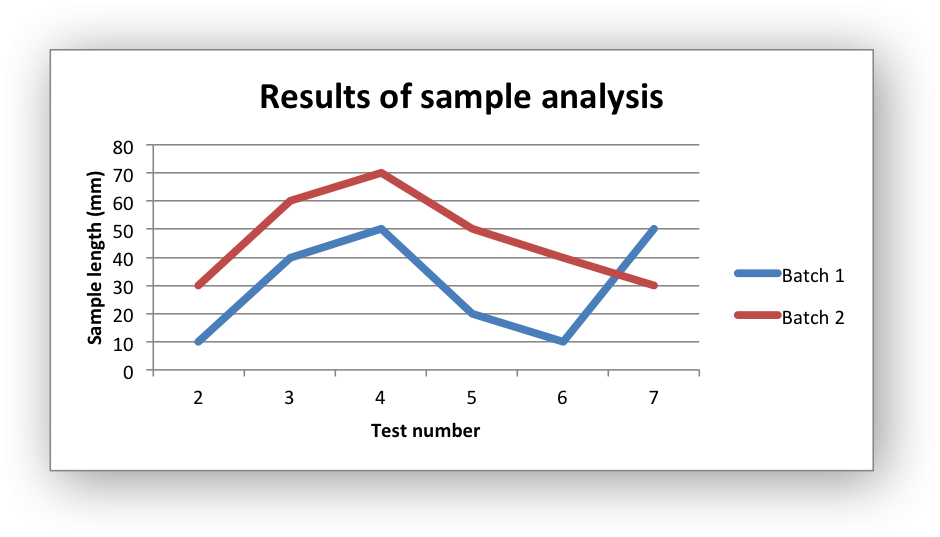# Example: Line Chart

Example of creating an Excel line chart. The X axis of a line chart is a category axis with fixed point spacing. For a line chart with arbitrary point spacing see the Scatter chart type.

Chart 1 in the following example is:```#######################################################################
#
# An example of creating Excel Line charts with Python and XlsxWriter.
#
# Copyright 2013-2019, John McNamara, jmcnamara@cpan.org
#
import xlsxwriter

workbook = xlsxwriter.Workbook('chart_line.xlsx')

# Add the worksheet data that the charts will refer to.
headings = ['Number', 'Batch 1', 'Batch 2']
data = [
[2, 3, 4, 5, 6, 7],
[10, 40, 50, 20, 10, 50],
[30, 60, 70, 50, 40, 30],
]

worksheet.write_column('A2', data)
worksheet.write_column('B2', data)
worksheet.write_column('C2', data)

# Create a new chart object. In this case an embedded chart.

# Configure the first series.
'name':       '=Sheet1!\$B\$1',
'categories': '=Sheet1!\$A\$2:\$A\$7',
'values':     '=Sheet1!\$B\$2:\$B\$7',
})

# Configure second series. Note use of alternative syntax to define ranges.
'name':       ['Sheet1', 0, 2],
'categories': ['Sheet1', 1, 0, 6, 0],
'values':     ['Sheet1', 1, 2, 6, 2],
})

# Add a chart title and some axis labels.
chart1.set_title ({'name': 'Results of sample analysis'})
chart1.set_x_axis({'name': 'Test number'})
chart1.set_y_axis({'name': 'Sample length (mm)'})

# Set an Excel chart style. Colors with white outline and shadow.
chart1.set_style(10)

# Insert the chart into the worksheet (with an offset).
worksheet.insert_chart('D2', chart1, {'x_offset': 25, 'y_offset': 10})

workbook.close()
```